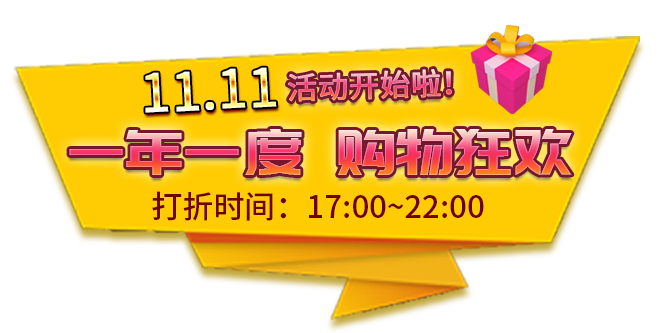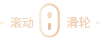风之武士(男) 防护服（男） 保卫者(男) 铸匠师(男) 风之武士(女) 防护服（女） 保卫者(女) 铸匠师(女) 疯狂科学家（男） 白狼刺客（男 街舞风行(男) 跆拳道少年(男) 疯狂科学家（女） 红莲忍者（女） 街舞风行(女) 跆拳道少女(女) 条纹时尚（男） 长款羽绒服（男） 顽皮企鹅装(男) 猎手(男) 条纹时尚（女） 长款羽绒服（女） 顽皮企鹅装(女) 猎手(女) 青甲神武（男） 机长（男） 朋克一族(男) 丛林游侠(男) 青甲神武（女） 空姐（女） 朋克一族(女) 丛林游侠(女) 私家侦探（男） 赛车达人（男） 冬日暖阳(男) 天之骄子(男) 私家侦探（女） 赛车女郎（女） 冬日暖阳(女) 千娇百媚(女) 旅行者（男） 飞行空军（男） 摄影师(男) 趣味猫咪装(男) 旅行者（女） 飞行空军（女） 摄影师(女) 趣味猫咪装(女) 特色秋装（男） 田园管主（男） 紫色西装(男) 锁甲斗士(男) 特色秋装（女） 田园管主（女） 紫色西装(女) 锁甲斗士(女) 私人管家（男） 因纽特（男） 练习生(男) 收割者(男) 私人管家（女） 因纽特（女） 练习生(女) 收割者(女) 热血青年（男） 网络红人（男） 炫彩花衣(男) 荒原游侠(男) 热血青年（女） 网络红人（女） 炫彩花裙(女) 荒原游侠(女) 暗影刺客（男） 部落风采（男） 垂钓爱好者(男) 骠骑将军(男) 暗影刺客（女） 部落风采（女） 垂钓爱好者(女) 骠骑将军(女) 特种军官（男） 炎龙风衣（男） 小当家(男) 夏日海风(男) 特种军官（女） 炎龙风衣（女） 小厨娘(女) 夏日海风(女) 午夜风行（男） 泰式风情（男） 网球教练(男) 人参娃娃(男) 午夜风行（女） 泰式风情（女） 网球教练(女) 人参娃娃(女) 街头少年（男） 希腊风情（男） 魔导师(男) 炼钢师(男) 嘻哈少女（女） 希腊风情（女） 魔导师(女) 炼钢师(女) 汉寿亭侯（男） 魔术师（男） 园艺师(男) 魅力歌星(男) 武圣后裔（女） 魔术师（女） 园艺师(女) 魅力歌星(女) 杏黄风衣（男） 闪烁之星(男) 咖啡师(男) 段雨(男) 藏蓝大衣（女） 闪烁之星(女) 咖啡师(女) 向晴(女) 兔男孩（男） 丞相(男) 悠闲时光(男) 舞动灵魂(男) 兔女郎（女） 丞相夫人(女) 悠闲时光(女) 舞动灵魂(女) 薄荷巧克力(男) 薄荷巧克力(女)